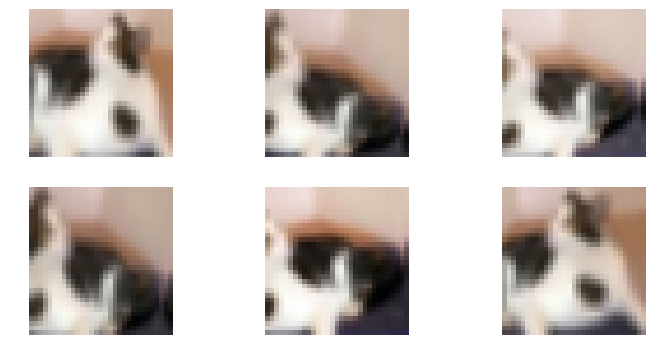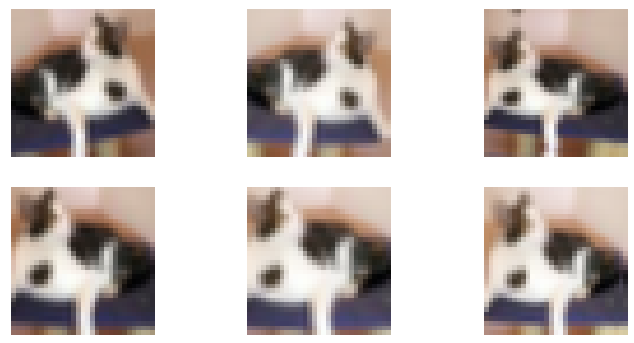# Test Time Augmentation (TTA) gives me a lower accuracy

I wanted to practice by creating a CIFAR10 classifier. In this notebook, I finetuned the CIFAR10 dataset with images of increasing size.

I wanted to try the test time augmentation to see how well my model was doing, like so:

``````ys,y = learn.TTA()
accuracy(ys, y)
``````

However, it appears to give me a lower accuracy (0.929 vs 0.935) than the model on its own, can it happen ? I remember from the course of last year that using TTA after training the model gave a significant increase in accuracy.

My hypothesis is that, as I work with pretty small images, data augmentation has a lot of influence on them, and can reduce the accuracy of the model. Maybe am I doing something wrong or missing something ?

Here is my notebook.

Edit : it appears that the default value of `scale` in `learn.TTA()`is too high for my usecase, I tried:

``````ys, y = learn.TTA(scale=1.1)
``````

and got an accuracy of 0.963. This could support my hypothesis.

6 Likes

That’s a really interesting result!Nice experiment.

1 Like

Thank you Jeremy !How would you explain it ? The padding ? (would make sense if a zoom out is performed but don’t know if it is the case)

cool~0.96 is a good score on cirfar10, and you can compare the results of different scales in your notebook。

See if you can figure out how to visualize the data augmentation by plotting a view examples of a single training image. That way you can see exactly what’s going on!1 Like

I repeated the experiment. Similarly, the default TTA value makes the predicted value worse.(0.935 vs 0.937)
The following is a comparison of the results of different scale values：

``````TTA(scale=1.05)   -> 0.9438
TTA(scale=1.10)   -> 0.9459
TTA(scale=1.15)   -> 0.9444
TTA(scale=1.20)   -> 0.9439
TTA(scale=1.25)   -> 0.9413
TTA(scale=1.35)   -> 0.9352  default
``````

The scale value is 1.1, TTA has the best result on cifar10
but I didn’t get the result of 0.96 through TTA. Is there any other tricks?

That is weird, I also did the same experiments yesterday and had those results:

``````TTA(scale=1.05)   -> 0.9632
TTA(scale=1.10)   -> 0.9629
TTA(scale=1.25)   -> 0.9569
TTA(scale=1.35)   -> 0.9294  default
``````

But I also tried:

`````` TTA(scale=1.)   -> 0.9625
TTA(scale=.95)   -> 0.9621
``````

Don’t know why you have different values, the gist is exactly what I did. Double check your learning rates through the notebook with the `lr_finder`, maybe they can slightly change from mine.

Here is what I came up with !

The default TTA gives this kind of images :And by changing the `scale` value, I have this :It now seems obvious why the default value leads to a lower accuracy, it even took me a while to understand what was on the first batch of picturesIt was also the first time I really dug into fastai source code, so I hope I have correctly understood and interpretated it (nice experience btw, I will do it again)

8 Likes